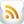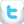## Calculating total and load capacities varying seating configurations

Calculate the available load capacity as shown in the following examples:

Example 1A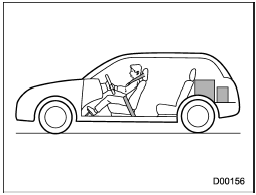Vehicle capacity weight of the vehicle is 900 lbs (408 kg), which is indicated on the vehicle placard with the statement “The combined weight of occupants and cargo should never exceed 900 lbs or 408 kg”.

For example, if the vehicle has one occupant weighing 154 lbs (70 kg) plus cargo weighing 662 lbs (300 kg).

1. Calculate the total weight.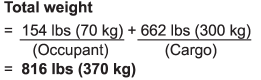2. Calculate the available load capacity by subtracting the total weight from the vehicle capacity weight of 900 lbs (408 kg).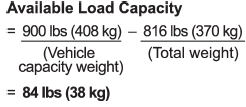3. The result of step 2 shows that a further 84 lbs (38 kg) of cargo can be carried.

Example 1B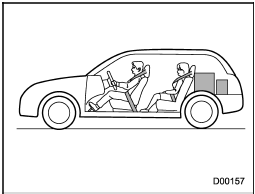For example, if a person weighing 176 lbs (80 kg) now enters the same vehicle (bringing the number of occupants to two), the calculations are as follows.

1. Calculate the total weight.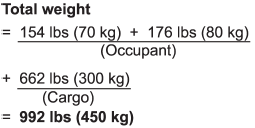2. Calculate the available load capacity.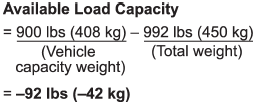3. The total weight now exceeds the capacity weight by 92 lbs (42 kg), so the cargo weight must be reduced by 92 lbs (42 kg) or more.

Example 2A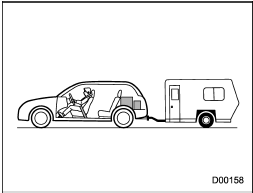Vehicle capacity weight of the vehicle is 900 lbs (408 kg), which is indicated on the vehicle placard with the statement “The combined weight of occupants and cargo should never exceed 408 kg or 900 lbs”.

For example, the vehicle has one occupant weighing 165 lbs (75 kg) plus cargo weighing 375 lbs (170 kg). In addition, the vehicle is fitted with a trailer hitch weighing 22 lbs (10 kg), to which is attached a trailer weighing 1,764 lbs (800 kg).

10% of the trailer weight is applied to the trailer tongue (i.e. Tongue load = 176 lbs (80 kg)).

1. Calculate the total weight.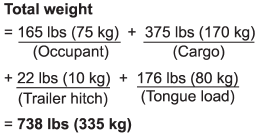2. Calculate the available load capacity.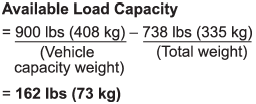3. The result of step 2 shows that a further 162 lbs (73 kg) of cargo can be carried.

NOTE
Your vehicle is neither designed nor intended to be used for trailer towing. Therefore, never tow a trailer with your vehicle.

Example 2B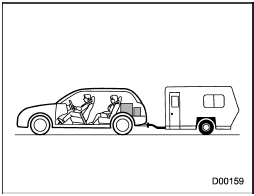For example, if a person weighing 143 lbs (65 kg) and a child weighing 40 lbs (18 kg) now enter the same vehicle (bringing the number of occupants to three), and a child restraint system weighing 11 lbs (5 kg) is installed in the vehicle for the child to use, the calculations are as follows.

1. Calculate the total weight.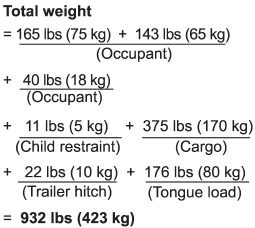2. Calculate the available load capacity.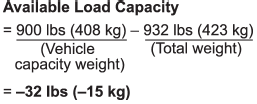3. The total weight now exceeds the capacity weight by 32 lbs (15 kg), so the cargo weight must be reduced by 32 lbs (15 kg) or more.

NOTE
Your vehicle is neither designed nor intended to be used for trailer towing. Therefore, never tow a trailer with your vehicle.